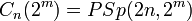# Chevalley group of type B

Jump to: navigation, search

## Definition

Let$K$ be a field and$n$ be a natural number. The Chevalley group of type B, denoted$B_n(K)$, is defined as follows:

1. Start with a symmetric bilinear form on$K^{2n + 1}$ under which the vector space decomposes as the sum of a$2n$-dimensional hyperbolic space and a one-dimensional anisotropic space. Note that for a finite field, this is the unique (up to equivalence) nondegenerate symmetric bilinear form on$K^{2n+1}$.
2. Consider the orthogonal group for this bilinear form.
3. Take the intersection of the kernels of the spinor norm and Dickson invariant maps (for characteristic not equal to 2, we could take the kernel of the determinant map instead of the kernel of the Dickson invariant map).

The group obtained at the end of Step (3) is the group$B_n(K)$. It is also denoted$\Omega_{2n+1}(K)$.

For a prime power$q$, we denote by$B_n(q)$ the group$B_n(\mathbb{F}_q)$.

## Collisions with other Chevalley groups

•$B_1(K)$ is always isomorphic to$A_1(K)$, i.e., the group$PSL(2,K)$.
• For characteristic two, these coincide with the projective symplectic groups. Specifically,$B_n(2^m) \cong C_n(2^m)$, where$C_n(2^m) = PSp(2n,2^m)$., Thus, for instance,$B_3(2^m)$ is isomorphic to$PSp(6,2^m)$.